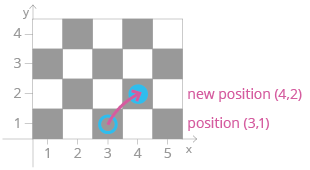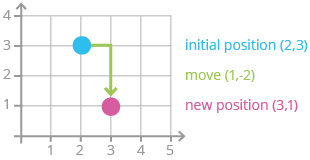Deals Of The Week - hours only!Up to 80% off on all courses and bundles.-Close
Recap
Tuples as return types in functions
Tuples as records
8. Tuples representing states or positions – coordinates
List of tuples
Summary

## Instruction

Very good! Apart from real-world objects, tuples can also be used to describe a given "state" or "position". For instance, they can represent a position in the Cartesian (x/y) coordinate system. For example, we can represent positions on various game boards. In the picture below, we represent the position of a red piece in a game of draughts (a.k.a. checkers) as (3, 1):

The red piece can move diagonally to the left or right, as shown in the example below:Let's try to write a function related to this movement. The function below accepts the current position as a tuple in the (x, y) format and returns the new position after a diagonal move to the right:

def move_up_right(current_position):
x, y = current_position
if x < 8 and y < 8:
return (x + 1, y + 1)
else:
return (x, y)

Note that we only make the move if the piece is not at the border of the board.

## Exercise

Write a function named get_new_position(position, move) which accepts two arguments: (1) the current position in the x/y coordinate system, and (2) the movement that was made.

Both arguments are given as two-element tuples, where the first element is the x value and the second element is the y value. Your task is to return the new position. Example:

for position = (2, 3) and move = (1, -2), the function should return (3, 1), as shown in the picture below:### Stuck? Here's a hint!

The task may seem complicated, but it is actually very simple! All you need to do is return the following tuple:

(position + move, position + move)